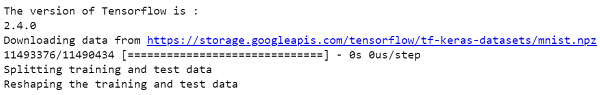# How can Tensorflow be used to save and load weights for MNIST dataset?

Tensorflow is a machine learning framework that is provided by Google. It is an open−source framework used in conjunction with Python to implement algorithms, deep learning applications and much more. It is used in research and for production purposes. It has optimization techniques that help in performing complicated mathematical operations quickly. This is because it uses NumPy and multi−dimensional arrays. These multi−dimensional arrays are also known as ‘tensors’.

The ‘tensorflow’ package can be installed on Windows using the below line of code −

pip install tensorflow

Tensor is a data structure used in TensorFlow. It helps connect edges in a flow diagram. This flow diagram is known as the ‘Data flow graph’. Tensors are nothing but multidimensional array or a list.

When the training occurs for long periods of time, the model tends to overfit and doesn’t generalize well on test data. Hence, the number of training steps has to be well-balanced. This means, all cases of data has to be taken to perform effective training. This way, the model generalizes better on test data. Otherwise, regularization can be performed.

Keras is a deep learning API, which is written in Python. It is a high−level API that has a productive interface that helps solve machine learning problems. It runs on top of Tensorflow framework. It was built to help experiment in a quick manner. It provides essential abstractions and building blocks that are essential in developing and encapsulating machine learning solutions.

Keras is already present within the Tensorflow package. It can be accessed using the below line of code.

import tensorflow
from tensorflow import keras

We are using the Google Colaboratory to run the below code. Google Colab or Colaboratory helps run Python code over the browser and requires zero configuration and free access to GPUs (Graphical Processing Units). Colaboratory has been built on top of Jupyter Notebook. Following is the code snippet −

## Example

!pip install -q pyyaml h5py
import os

import tensorflow as tf
from tensorflow import keras

print("The version of Tensorflow is : ")
print(tf.version.VERSION)
(train_images, train_labels), (test_images, test_labels) = tf.keras.datasets.mnist.load_data()
print("Splitting training and test data")
train_labels = train_labels[:1000]
test_labels = test_labels[:1000]

print("Reshaping the training and test data")
train_images = train_images[:1000].reshape(-1, 28 * 28) / 255.0
test_images = test_images[:1000].reshape(-1, 28 * 28) / 255.0

## Output## Explanation

• Import the required packages and alias them.

• Get the first 1000 examples to improve the speed of execution.